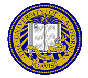# Lecture 12: October 21, 2019

Due: Lab 1, due October 18, 2019; Homework 2, due October 21, 2019

1. Greetings and felicitations!
2. Puzzle of the Day
3. Symmetric Cryptography
1. Polyalphabetic: Vigenère, fi(a) = a + ki mod n
2. Cryptanalysis: first do index of coincidence to see if it is monoalphabetic or polyalphabetic, then Kasiski method.
3. Problem: eliminate periodicity of key
4. Long key generation
1. Autokey cipher: key is keyword followed by plaintext or cipher text
2. Running-key cipher: key is simply text; wedge is that (plaintext, key) letter pairs are not random (T/T, H/H, E/E, T/S, R/E, A/O, S/N, etc.)
3. Perfect secrecy: when the probability of computing the plaintext message is the same whether or not you have the ciphertext; only cipher with perfect secrecy: one-time pads; C = AZPC; is that DOIT or DONT?
5. Product ciphers
1. DES
2. AES
6. Public-Key Cryptography
1. Basic idea: 2 keys, one private, one public
2. Cryptosystem must satisfy:
1. Given public key, computationally infeasible to get private key;
2. Cipher withstands chosen plaintext attack;
3. Encryption, decryption computationally feasible (note: commutativity not required)
3. Benefits: can give confidentiality or authentication or both
7. Use of public key cryptosystem
1. Normally used as key interchange system to exchange secret keys (cheap)
2. Then use secret key system (too expensive to use public key cryptosystem for this)
8. RSA
1. Provides both authenticity and confidentiality
2. Go through algorithm:
Idea: C = Me mod n, M = Cd mod n, with ed mod φ(n) = 1
Public key is (e, n); private key is d. Choose n = pq; then φ(n) = (p−1)(q−1).
3. Example: p = 5, q = 7; then n = 35, φ(n) = (5−1)(7−1) = 24. Pick d = 11.
Then ed mod φ(n) = 1, so e = 11
To encipher 2, C = Me mod n = 211 mod 35 = 2048 mod 35 = 18, and M = Cd mod n = 1811 mod 35 = 2.
4. Example: p = 53, q = 61; then n = 3233, φ(n) = (53−1)(61−1) = 3120. Pick d = 791. Then e = 71
To encipher M = RENAISSANCE, use the mapping A = 00, B = 01, …, Z = 25, (space) = 26.
Then: M = RE NA IS SA NC E␢ = 1704 1300 0818 1800 1302 0426
So: C = 170471 mod 3233 = 3106; … = 3106 0100 0931 2691 1984 2927Matt Bishop Office: 2209 Watershed Sciences Phone: +1 (530) 752-8060 Email: mabishop@ucdavis.edu
ECS 153, Computer Security
Version of October 18, 2019 at 11:57PM